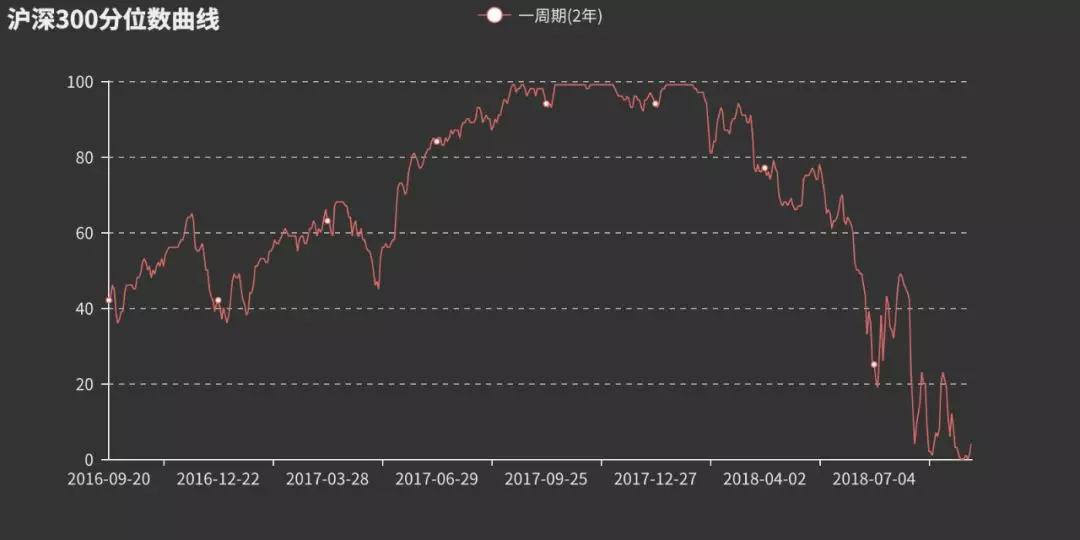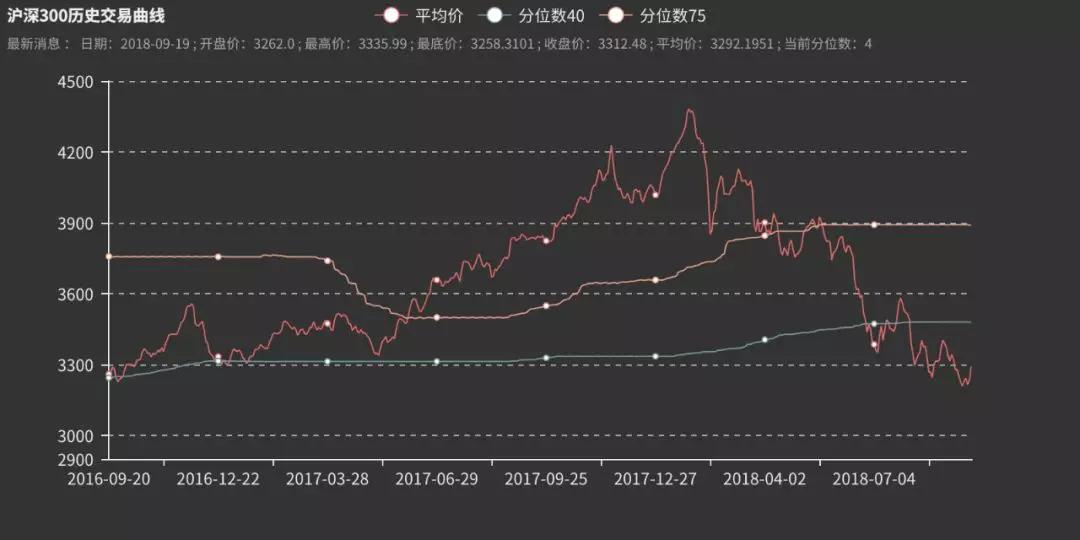# 如何利用Python開發一套股票分析軟件

2019-06-24 20:34:57 看盤

【1】分析網址獲得 HTML

（下面代碼我們默認股票代碼為 399300，代碼中year和season為參數，返回頁面的 HTML，存放在 list 中。要獲得多年的數據，可寫一個 for 循環來循環爬取網頁内容，這裡就不做過多展示了。)

def __init__(self, year, season):

self.year = year

self.season  = season

self.soup = None

def SpiderData(self):

list = []

headers = {'User-Agent': 'Mozilla/5.0 (X11; Ubuntu; Linux x86_64; rv:62.0) Gecko/2010010 Firefox/62.0'}

temp = url + str(self.year) + '&season=' + str(self.season)

self.soup = BeautifulSoup(html, 'lxml')

self.Spider(list)

return list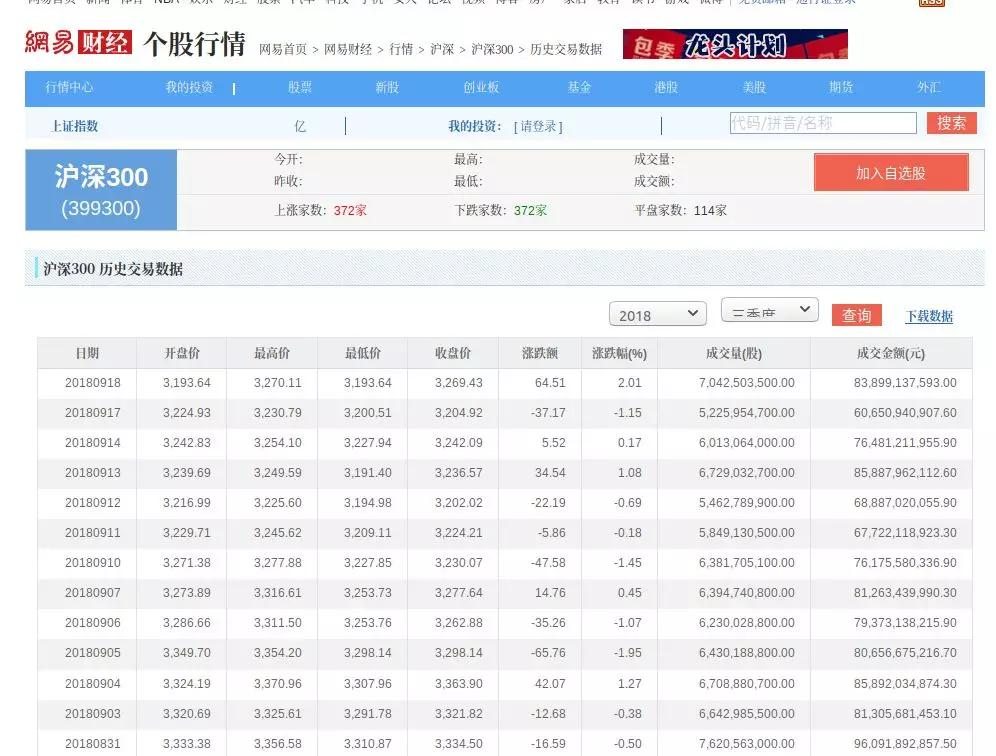【2】 從HTML中拿到我們需要的數據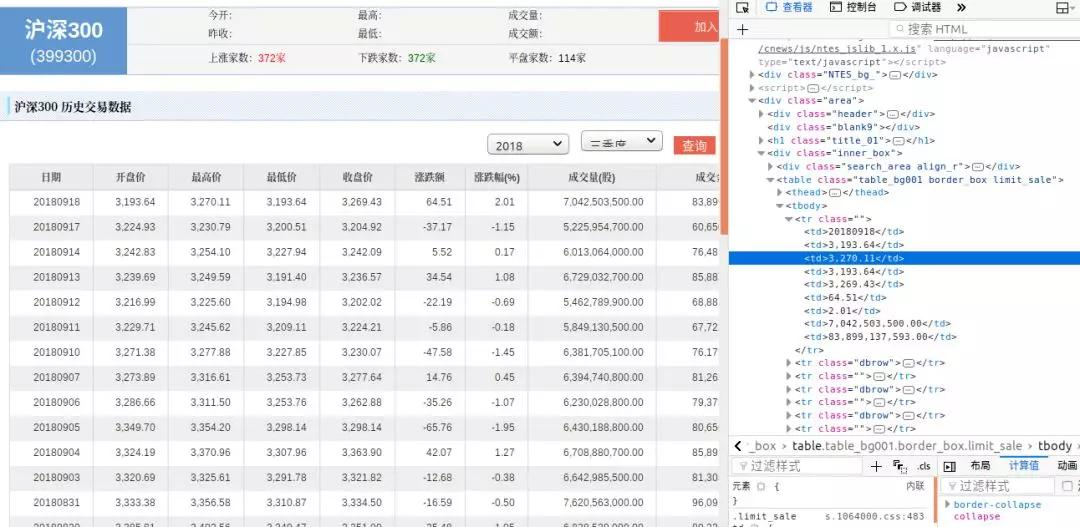BeautifulSoup 中文官網如下：

• https://www.crummy.com/software/BeautifulSoup/bs4/doc.zh/

def Spider(self,list):

tag = self.soup.find('table', {'class': "table_bg001 border_box limit_sale"})

data = tag.find_all('td')

for i in data:

list.append(i.text)

【1】數據分析包括兩塊：

• 第一是計算滬深300交易數據的分位數；
• 第二是數據的讀取與更新

• https://zh.wikipedia.org/wiki/Percentile

【2】有了算法，我們就開始處理數據。我們要從數據庫中獲得我們需要的數據，而我們在計算分位數時面對的是平均數（收盤價、開盤價以及最高價、最低價的平均數），故應該先計算出平均數，然後算出分位數。

def GetFra(self):

for i in self.date:

sql = "SELECT Average_One FROM stock WHERE Date BETWEEN DATE_SUB('" + str(

i) + "' ,INTERVAL " + str(self.period) + " YEAR) AND '" + str(i) + "'ORDER BY Average_One ASC;"

days = self.cursor.execute(sql)

list = self.cursor.fetchall()

sql1 = "SELECT Average_one FROM stock WHERE Date = '"+str(i)+"';"

self.cursor.execute(sql1)

temp = self.cursor.fetchone()

sql2 = "UPDATE stock SET Fractile_Now=" + str(

(list.index(temp)) * 100 // days) + " WHERE Date='" + str(i) + "';"

self.cursor.execute(sql2)

self.db.commit()

self.listone.append(numpy.percentile(list, 25))

def Average(self):

sql = "UPDATE stock SET Average_One=(Open_price+Top_price+Floor_price+Close_price)*0.25;"

self.cursor.execute(sql)

self.cursor.execute(sql1)

self.db.commit()

Python 給我們提供了計算分位數的簡便方法，一個是 list 的各種操作，通過它的index方法可以計算當前分為數；另一個就是numpy ，numpy.percentile可以迅速對 list 或者元組排序，然後得到分位數，是不是很方便。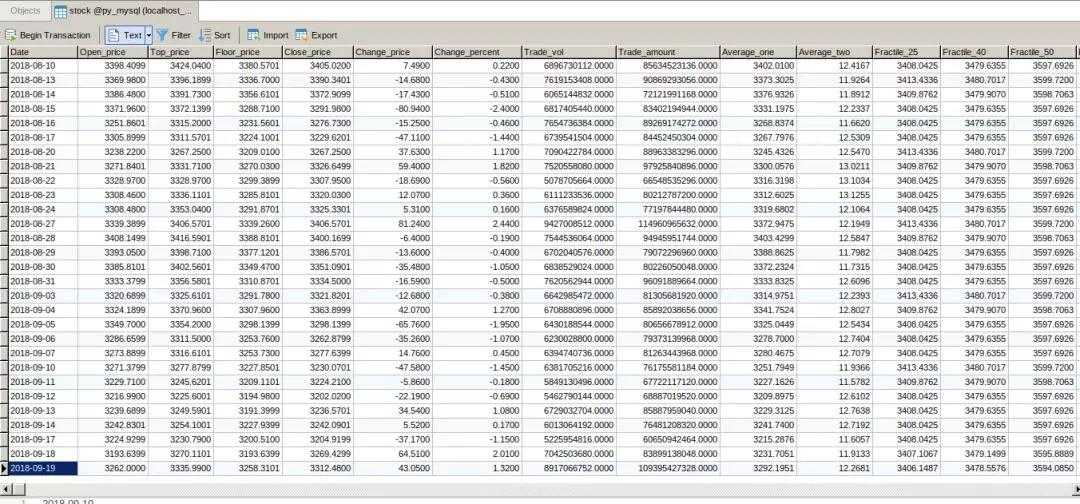• https://www.navicat.com/en/products

【3】在數據的可視化之前，我們還要解決數據的讀取與更新問題，數據的讀取很好弄，cursor.fetall會返回一個元組，我們通過 sql 語句就可以解決數據的讀取問題；而更新操作就沒有那麼容易，網頁上股票數據除了節假日都會更新，我們需要每天定時爬取數據以及處理數據，作者通過使用的 ubuntu 的crontab 定時運行 python 腳本文件，具體實施大家可以找度娘，因為不同電腦環境配置不同，出現的問題也比較複雜，作者能力有限，就不提供具體方法了。

【1】數據的可視化還是比較容易的，因為我們的數據庫中已經處理好了數據，無論是每天的平均價格還是分位數都在數據庫中，我們隻需要提取數據，然後作圖就好了。

【2】在作圖上，作者使用的是pyecharts，不過要pyecharts做出來的圖需要在浏覽器上打開，當然，還有pyecharts不會這麼水，在搭建了Nodejs後，使用pyecharts-snapshot插件就可以在指定位置生成圖片了。具體pyecharts教程參考鍊接：

• http://pyecharts.org/#/zh-cn/prepare

【3】可視化就介紹這些，下面看代碼：

db = pymysql.connect('localhost', 'root', '******', '****')

cursor = db.cursor()

sql_75 = "SELECT Fractile_75 FROM stock WHERE Date BETWEEN  DATE_SUB('" +str(self.time) + "' ,INTERVAL 2 YEAR)  AND '" + str(self.time) + "' ORDER BY DATE ASC;"

sql_40 = "SELECT Fractile_40 FROM stock WHERE Date BETWEEN  DATE_SUB('" +str(self.time) + "' ,INTERVAL 2 YEAR)  AND '" + str(self.time) + "' ORDER BY DATE ASC;"

sql_average = "SELECT Average_one FROM stock WHERE Date BETWEEN  DATE_SUB('" +str(self.time) + "' ,INTERVAL 2 YEAR)  AND '" + str(self.time) + "' ORDER BY DATE ASC;"

sql1 = "SELECT Date FROM stock WHERE Date BETWEEN  DATE_SUB('" +str(self.time) + "' ,INTERVAL 2 YEAR)  AND '" + str(self.time) + "' ORDER BY DATE ASC;"

cursor.execute(sql_75)

data_75 = cursor.fetchall()

cursor.execute(sql_40)

data_40 = cursor.fetchall()

cursor.execute(sql_average)

data_average = cursor.fetchall()

top = max(data_average)- 300

floor = min(data_average)- 300

cursor.execute(sql1)

date = cursor.fetchall()

temp = []

for i in date:

temp.append(i)

line1 = Line("滬深300曆史交易曲線", self.text ,title_text_size=12 ,subtitle_text_size=10 )

line1.use_theme("dark")   #設置背景

line1.add("平均價", temp, data_average, ys_aximin=math.floor(floor/100)*100, ys_aximax=math.ceil(top/100)*100)

line1.add("分位數40", temp, data_40 ,yaxis_min=math.floor(floor/100)*100, ys_aximax=math.ceil(top/100)*100)

line1.add("分位數75", temp, data_75,yaxis_min=math.floor(floor/100)*100 ,ys_aximax=math.ceil(top/100)*100)